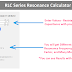In this RLC Series Resonance Circuit Calculator, you can calculate Resonant Frequency(f0), Bandwidth(b), Upper Cut-off Frequency(fH), Lower Cut-off Frequency(fL), Q factor(Q), Damping(d), Impedance(Z) and many more Parameters.

RLC Series Resonance Calculator

## RLC Series Resonance Calculator

### Calculated Values:

Resonant Frequency f0: ()

Bandwidth b: ()

Upper Cut-off fH: ()

Lower Cut-off fL: ()

Reactance in the Circuit XL = XC: ()

Impedance Z: ()

Total Current Flow I: ()

Voltage Across Inductor and Capacitor VX: ()

Voltage Across Resistor VR: ()

Q factor Q:

Damping d:

### How to Use This Calculator?

To use the above "RLC Series Resonance Calculator," follow the below steps,

1. Enter the value of the inductor (L) in the first input field. Select the appropriate unit from the dropdown menu.

2. Enter the value of the capacitor (C) in the second input field. Select the appropriate unit from the dropdown menu.

3. Enter the value of the resistor (R) in the third input field. Select the appropriate unit from the dropdown menu.

4. Enter the value of the voltage (U) in the fourth input field. Select the appropriate unit from the dropdown menu.

5. After filling in all the required values, click on the "Calculate" button at the bottom of the form.

6. Once you click on the "Calculate" button, the calculator will process the input data and display the values of different parameters of an RLC Series Resonance Circuit.

### Formula

Here are the formulas, the calculator uses to calculate different parameters,

Resonant Frequency Formula:

resonant frequency = 1 / (2 * π * √(inductor * capacitor))

Reactance in the Circuit Formula:

reactance = 2 * π * resonant frequency * inductor

Impedance Formula:

impedance = resistor

Total Current Flow Formula:

total current = voltage/impedance

Voltage Across Inductor and Capacitor Formula:

VX = total current * reactance

Voltage Across Resistor Formula:

VR = total current * resistance

Q Factor Formula:

Q factor = reactance/resistance

Damping Factor Formula:

Damping(D) = 1 / Q factor

Bandwidth Formula:

bandwidth = resonant frequency / Q Factor

Upper Cut-off Frequency Formula:

fH = resonant frequency + (bandwidth / 2)

Lower Cut-off Frequency Formula:

fL = resonant frequency - (bandwidth / 2)

### What is the RLC Series Resonance Circuit?

An RLC Series Resonance circuit is also known as a series resonant circuit. It is an electrical circuit that consists of three passive components connected in series such as a resistor (R), an inductor (L), and a capacitor (C). In this circuit configuration, the components are connected end-to-end which forms a single closed loop.

At the resonant frequency, the reactance of the inductor (XL) is almost equal to the reactance of the capacitor (XC), which causes a minimum impedance. In this condition, the circuit becomes purely resistive, and the current flow is at its maximum.

RLC Series Resonance circuits are used in various applications such as filter design, communication systems, and tuning circuits. Understanding the behavior of these circuits is essential in electronics and electrical engineering.

Thank you for visiting the Website. Keep visiting for more Updates.

RLC Series Resonance Calculator (Frequency, Bandwidth, and More)Reviewed by Author on July 26, 2023 Rating: 5